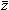Cavity method for force transmission in jammed disordered packings of hard particles

Author affiliations

Abstract

The force distribution of jammed disordered packings has always been considered a central object in the physics of granular materials. However, many of its features are poorly understood. In particular, analytic relations to other key macroscopic properties of jammed matter, such as the contact network and its coordination number, are still lacking. Here we develop a mean-field theory for this problem, based on the consideration of the contact network as a random graph where the force transmission becomes a constraint satisfaction problem. We can thus use the cavity method developed in the past few decades within the statistical physics of spin glasses and hard computer science problems. This method allows us to compute the force distribution P(f) for random packings of hard particles of any shape, with or without friction. We find a new signature of jamming in the small force behavior P(f) ∼ fθ, whose exponent has attracted recent active interest: we find a finite value for P(f = 0), along with θ = 0. Furthermore, we relate the force distribution to a lower bound of the average coordination numberminc(μ) of jammed packings of frictional spheres with coefficient μ. This bridges the gap between the two known isostatic limitsc (μ = 0) = 2D (in dimension D) andc(μ → ∞) = D + 1 by extending the naive Maxwell's counting argument to frictional spheres. The theoretical framework describes different types of systems, such as non-spherical objects in arbitrary dimensions, providing a common mean-field scenario to investigate force transmission, contact networks and coordination numbers of jammed disordered packings.Publication details

The article was received on 27 Mar 2014, accepted on 03 Jun 2014 and first published on 06 Jun 2014

Article type: Paper
DOI: 10.1039/C4SM00667D
Soft Matter, 2014,10, 7379-7392

•Cavity method for force transmission in jammed disordered packings of hard particles

L. Bo, R. Mari, C. Song and H. A. Makse, Soft Matter, 2014, 10, 7379
DOI: 10.1039/C4SM00667D